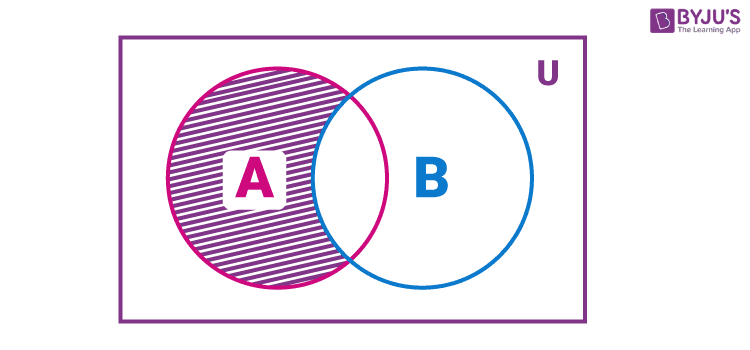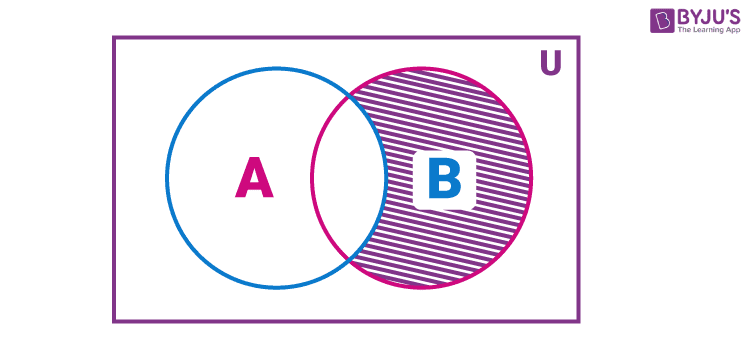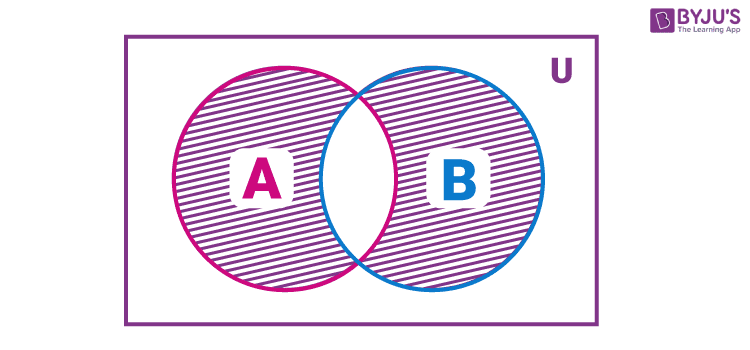## Subject: Elementary Mathematics

Q.1 Define difference and symmetric difference of two sets and prove that .
(A− B)U(B − A) = (AUB)−(A∩ B)

## Difference of Two Sets :

The difference of the sets A and B in this order is the set of elements which belong to A but not to B. Symbolically, we write A – B and read as “ A minus B”.

The representation of A – B using a Venn diagram is given below.Similarly, we can find B – A, the difference of the sets B and A in this order is the set of elements which belong to B but not to A. Symbolically, we write B – A and read as “ B minus A”. and a Venn diagram for B – A as:Also, note that A – B is not equal to B – A, i.e. A – B ≠ B – A.

### Symmetric Difference between Two Sets:

The set which contains the elements which are either in set A or in set B but not in both is called the symmetric difference between two given sets. It is represented by A ⊝ B and is read as a symmetric difference of set A and B.We have to prove that : (A-B) U (B-A) = (A∪B) – (A∩B)

Proof:

Let, x ∈ (A-B) U (B-A)

⇒ x ∈ (A-B) or x ∈ (B-A)

⇒ x ∈ A But x ∉ B or x ∈ B but x ∉ A,

⇒ x ∈ A or x ∈ B

⇒ x ∈ (A∪B)

⇒ x ∈ (A∪B) – (A∩B)

Since here x represents the arbitrary element of the set (A-B) U (B-A).

Thus, (A-B) U (B-A) = (A∪B) – (A∩B)

Q.2 If R is an equivalence relation in a set A , then Prove that R-1 is also an equivalence relation in A

Solution:

## An equivalence relation is one which is reflexive, symmetric and transitive. R is an equivalence relation on set A. Let the element of set A be

So, – since it is reflexive, this is also true for It is symmetric hence, and this is also true for Also, R is transitive i.e., and For and or Thus is symmetric, reflexive and transitive. i.e. is equivalence Relation.

Q.3 Find out the square root of complex numbers 12+5i

Solution :

√12–5i)=a+ib,

(a+ib)2=12–5i,

(a2−b2)+2abi=12–5i,

a2−b2=12, 2ab=−5,

(a2+b2)2=(a2−b2)2+(2ab)2

=122+52=144+25=169=132,

a2+b2=13,

2a2=12+13=25, 2b2=13−12=1,

a=±52–√, b=∓12–√, (ab<0)

a+ib=±12–√(5−i).